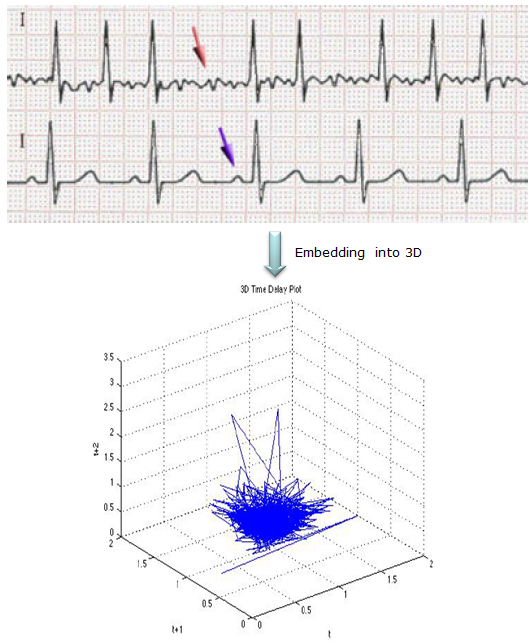Engineering Math - Quick Reference                                 Home : www.sharetechnote.com Chaos - Embedding   The defintion of Embedding here may sound a little differently from what you find in the dictionary. Simply put, Embedding in Chaos analysis is a method of grouping a set of sequence of data into a different format. Usually as a result of Embedding, we usually get a bunch of points in a coordinate system from the original data. In most case, the embedded data is displayed in the coordinate which has higher dimension than the orginal data.   A few examples are as follows.   Case 1 >   (A) is original data and (B) is the embedded data. (A) is just a sequence of numbers which can be labeled as a '1-D' data. In (B), we gets a bunch of coordinate of {(x0,x1),(x1,x2),..,(x(n-2),x(n-1))} which can be labeled as '2-D' data.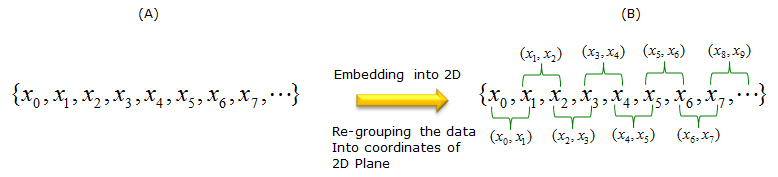Case 2 >   You can also embed as follows as well. In this case, '1-D' data is transformed (embedded) into '3-D' data.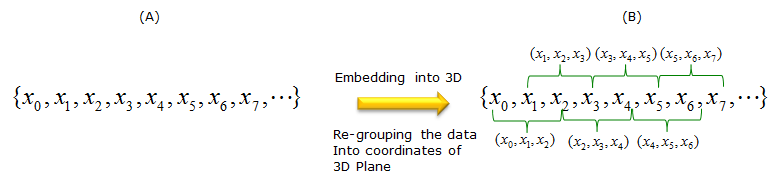Case 3 >   There is no single/fixed method for embedding. You can embed the data in any way that would give you the best information. For example, even in case of 1D to 2D embedding, you can group the data as follows. (Compare this to the first case). Again, this is not the only way, you can group the data in anyway as long as it gives you any meaningful result.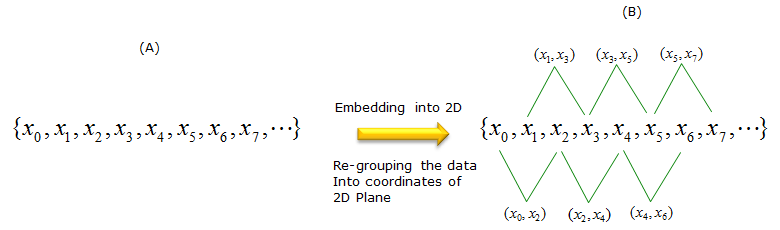Now you would have an important question. Why do we use this kind of method ? The answer is simple. It is because the embedded result would give you better / clear information (view) than the original data. Of course, you may come out with even more confusing result than the original data, but those embedding will be rejected by others (rejected even by yourself).   Following is one example. The graph on the left side is the plotting of an original data (the data before embedding). If you want to know how you get this data, see Logistics page. (B) is the result of Embedding based on < Case 1 > described above. I would not go into mathmetical details of this process, but you would see intuitively that (B) gives you much more clear understanding of the data comparing to graph (A).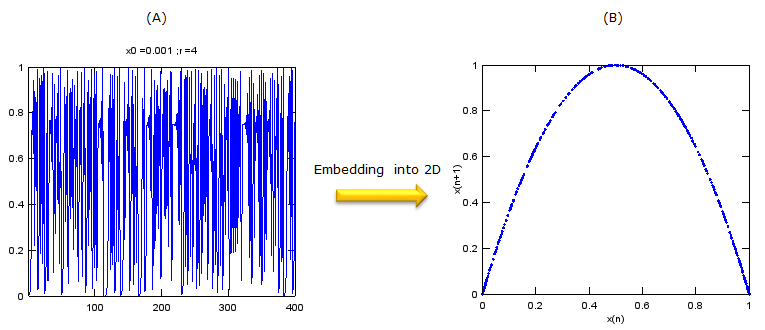Example 01 : EKG Analysis >   This is one example of using embedding technique (this is '1D' to '3D' embedding) which can be used in real engineering. You would see a 3D graph as the result of the process. You may not see a clear meaning of the resulting 3D graph, but there are some additional mathematical technique to extract more clearer meaning from the resulting embedded graph. I will post another pages about this additional mathematical technique when I find time.